pyclustering.utils.metric Namespace Reference

Module provides various distance metrics - abstraction of the notion of distance in a metric space. More...

## Classes

class  distance_metric
Distance metric performs distance calculation between two points in line with encapsulated function, for example, euclidean distance or chebyshev distance, or even user-defined. More...

class  type_metric
Enumeration of supported metrics in the module for distance calculation between two points. More...

## Functions

def euclidean_distance (point1, point2)
Calculate Euclidean distance between two vectors. More...

def euclidean_distance_numpy (object1, object2)
Calculate Euclidean distance between two objects using numpy. More...

def euclidean_distance_square (point1, point2)
Calculate square Euclidean distance between two vectors. More...

def euclidean_distance_square_numpy (object1, object2)
Calculate square Euclidean distance between two objects using numpy. More...

def manhattan_distance (point1, point2)
Calculate Manhattan distance between between two vectors. More...

def manhattan_distance_numpy (object1, object2)
Calculate Manhattan distance between two objects using numpy. More...

def chebyshev_distance (point1, point2)
Calculate Chebyshev distance between between two vectors. More...

def chebyshev_distance_numpy (object1, object2)
Calculate Chebyshev distance between two objects using numpy. More...

def minkowski_distance (point1, point2, degree=2)
Calculate Minkowski distance between two vectors. More...

def minkowski_distance_numpy (object1, object2, degree=2)
Calculate Minkowski distance between objects using numpy. More...

def canberra_distance (point1, point2)
Calculate Canberra distance between two vectors. More...

def canberra_distance_numpy (object1, object2)
Calculate Canberra distance between two objects using numpy. More...

def chi_square_distance (point1, point2)
Calculate Chi square distance between two vectors. More...

def chi_square_distance_numpy (object1, object2)
Calculate Chi square distance between two vectors using numpy. More...

## Detailed Description

Module provides various distance metrics - abstraction of the notion of distance in a metric space.

Date
2014-2019

## ◆ canberra_distance()

 def pyclustering.utils.metric.canberra_distance ( point1, point2 )

Calculate Canberra distance between two vectors.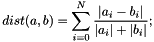Parameters
 [in] point1 (array_like): The first vector. [in] point2 (array_like): The second vector.
Returns
(float) Canberra distance between two objects.

Definition at line 465 of file metric.py.

## ◆ canberra_distance_numpy()

 def pyclustering.utils.metric.canberra_distance_numpy ( object1, object2 )

Calculate Canberra distance between two objects using numpy.

Parameters
 [in] object1 (array_like): The first vector. [in] object2 (array_like): The second vector.
Returns
(float) Canberra distance between two objects.

Definition at line 490 of file metric.py.

## ◆ chebyshev_distance()

 def pyclustering.utils.metric.chebyshev_distance ( point1, point2 )

Calculate Chebyshev distance between between two vectors.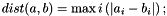Parameters
 [in] point1 (array_like): The first vector. [in] point2 (array_like): The second vector.
Returns
(double) Chebyshev distance between two vectors.
euclidean_distance_square, euclidean_distance, minkowski_distance

Definition at line 389 of file metric.py.

## ◆ chebyshev_distance_numpy()

 def pyclustering.utils.metric.chebyshev_distance_numpy ( object1, object2 )

Calculate Chebyshev distance between two objects using numpy.

Parameters
 [in] object1 (array_like): The first array_like object. [in] object2 (array_like): The second array_like object.
Returns
(double) Chebyshev distance between two objects.

Definition at line 414 of file metric.py.

## ◆ chi_square_distance()

 def pyclustering.utils.metric.chi_square_distance ( point1, point2 )

Calculate Chi square distance between two vectors.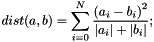Parameters
 [in] point1 (array_like): The first vector. [in] point2 (array_like): The second vector.
Returns
(float) Chi square distance between two objects.

Definition at line 509 of file metric.py.

## ◆ chi_square_distance_numpy()

 def pyclustering.utils.metric.chi_square_distance_numpy ( object1, object2 )

Calculate Chi square distance between two vectors using numpy.

Parameters
 [in] object1 (array_like): The first vector. [in] object2 (array_like): The second vector.
Returns
(float) Chi square distance between two objects.

Definition at line 534 of file metric.py.

## ◆ euclidean_distance()

 def pyclustering.utils.metric.euclidean_distance ( point1, point2 )

Calculate Euclidean distance between two vectors.

The Euclidean between vectors (points) a and b is calculated by following formula: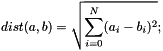Where N is a length of each vector.

Parameters
 [in] point1 (array_like): The first vector. [in] point2 (array_like): The second vector.
Returns
(double) Euclidean distance between two vectors.
euclidean_distance_square, manhattan_distance, chebyshev_distance

Definition at line 279 of file metric.py.

## ◆ euclidean_distance_numpy()

 def pyclustering.utils.metric.euclidean_distance_numpy ( object1, object2 )

Calculate Euclidean distance between two objects using numpy.

Parameters
 [in] object1 (array_like): The first array_like object. [in] object2 (array_like): The second array_like object.
Returns
(double) Euclidean distance between two objects.

Definition at line 302 of file metric.py.

## ◆ euclidean_distance_square()

 def pyclustering.utils.metric.euclidean_distance_square ( point1, point2 )

Calculate square Euclidean distance between two vectors.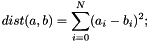Parameters
 [in] point1 (array_like): The first vector. [in] point2 (array_like): The second vector.
Returns
(double) Square Euclidean distance between two vectors.
euclidean_distance, manhattan_distance, chebyshev_distance

Definition at line 315 of file metric.py.

Referenced by pyclustering.utils.metric.euclidean_distance().

## ◆ euclidean_distance_square_numpy()

 def pyclustering.utils.metric.euclidean_distance_square_numpy ( object1, object2 )

Calculate square Euclidean distance between two objects using numpy.

Parameters
 [in] object1 (array_like): The first array_like object. [in] object2 (array_like): The second array_like object.
Returns
(double) Square Euclidean distance between two objects.

Definition at line 338 of file metric.py.

## ◆ manhattan_distance()

 def pyclustering.utils.metric.manhattan_distance ( point1, point2 )

Calculate Manhattan distance between between two vectors.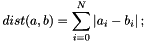Parameters
 [in] point1 (array_like): The first vector. [in] point2 (array_like): The second vector.
Returns
(double) Manhattan distance between two vectors.
euclidean_distance_square, euclidean_distance, chebyshev_distance

Definition at line 351 of file metric.py.

## ◆ manhattan_distance_numpy()

 def pyclustering.utils.metric.manhattan_distance_numpy ( object1, object2 )

Calculate Manhattan distance between two objects using numpy.

Parameters
 [in] object1 (array_like): The first array_like object. [in] object2 (array_like): The second array_like object.
Returns
(double) Manhattan distance between two objects.

Definition at line 376 of file metric.py.

## ◆ minkowski_distance()

 def pyclustering.utils.metric.minkowski_distance ( point1, point2, degree = 2 )

Calculate Minkowski distance between two vectors.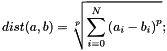Parameters
 [in] point1 (array_like): The first vector. [in] point2 (array_like): The second vector. [in] degree (numeric): Degree of that is used for Minkowski distance.
Returns
(double) Minkowski distance between two vectors.
euclidean_distance

Definition at line 427 of file metric.py.

## ◆ minkowski_distance_numpy()

 def pyclustering.utils.metric.minkowski_distance_numpy ( object1, object2, degree = 2 )

Calculate Minkowski distance between objects using numpy.

Parameters
 [in] object1 (array_like): The first array_like object. [in] object2 (array_like): The second array_like object. [in] degree (numeric): Degree of that is used for Minkowski distance.
Returns
(double) Minkowski distance between two object.

Definition at line 451 of file metric.py.